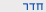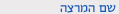שנה"ל תש"ףמשוואות דיפרנציאליות ואינטגרליות
Differential and Integral Equations
0510-5001-01
הנדסה | ביה"ס להנדסת חשמלסמ'  א' 0900-1200 'ו 001 ולפסון הנדסה שיעור פרופ מלמד בוריס הקורס מועבר באנגלית
ש"ס:  3.0

סילבוס מקוצר
משקל:                  3
משוואות דיפרנציאליות חלקיות מסדר ראשון: משוואות לינאריות, לינאריות למחצה ולא לינאריות. שיטת האופינים למשוואות לינאריות ולא לינאריות. משטח מוקדי caustic. משוואות דיפרנציאליות חלקיות מסדר שני וסיווגן: אליפטיות הפרבוליות ופרבוליות. אופיינים. בעיית קושי ויחידות הפתרון. בעיות תנאי שפה. אופרטורים דיפרנציאליים צמודים וצמודים לעצמם. משפט גרין. שיטת רימן. יחידות פתרון למשוואות אליפטיות צמודות לעצמן. משוואות אינטגרליות. סיווג. פונקציות גרין ופיתוח משוואות אינטגרליות בעזרתן. בעיות רבי ממדיות. שימושים של משפט גרין. תורת הפיזור. גרעינים פריקים, תיאוריית הילברט שמידט. שיטות איטרטיביות לפתרון משוואות אינטגרליות. שיטת גלרקין. חשבון וריאציוני: ניסוח בסיסי, משוואות אוילר. כופלי לגרנז', שיטת רילי-ריץ לפתרון משואות אינטגרליות.הנדסה | ביה"ס להנדסת חשמל
0510-5001-01 משוואות דיפרנציאליות ואינטגרליות
Differential and Integral Equations
שנה"ל תש"ף | סמ'  א' | פרופ מלמד בוריס

סילבוס מפורט/דף מידע

Differential and Integral Equations

Lecturer: Boris Melamed (Malomed)

Credits: 3

Updated syllabus (remark: if the semester is not long enough, some advanced items may be dropped)

The course may be taught in Hebrew or English. A full videorecord of the course taught in Hebrew is available at:

http://video.tau.ac.il/index.php?option=com_videos&Itemid=53&lang=en

Then select Faculty/Department: No. 0510,  חשמל להנדסת ב'ה''ס Then select course No. 5001, ואינטגרליות דיפרנצליות משוואות

Partial differential equations (PDEs): general introduction (it includes the derivation of the "master equation", namely, a generalized multidimensional sine-Gordon equation, from which a majority of classical PDEs, both linear and nonlinear ones, can be obtained as particular and limit cases). Classification of classical linear second-order

PDEs: elliptic, hyperbolic,

and parabolic equations; transformation of generic equations, belonging to this class, into canonical forms. A brief introduction to more sophisticated PDEs (Klein-Gordon, Helmholtz, Schroedinger, sine-Gordon, and Burgers equations). The initial-value (Cauchy) problem, and boundary-value problems (of the Dirichlet, Neumann, and mixed types). Stability of solutions and well-posedness of the PDEs. The solution of the Cauchy problem for the one-dimensional (1D) D'Alembert equation (traveling waves).  Solutions of Cauchy problem for the 1D diffusion equation in finite regions (with boundary conditions) in the form of Fourier series. The Green's function and solution of the Cauchy problem for the 1D diffusion  equation in the infinite domain. The Green's function for two- and three-dimensional (2D and 3D) Poisson equations, and an elementary introduction to the theory of renormalization for singular solutions (using the 2D Poisson equation as an example). Distributions (the Dirac's delta-function and related functions). Quasi-linear PDEs of the first order and the method of characteristics; the problem of caustics. The Burgers equation, shock waves, and the Rankine-Hugoniot relation, as the regularization of singular solutions of the quasi-linear equations. Introduction to nonlinear PDEs: the Korteweg - de Vries equation, nonlinear Schroedinger equation, and elementary soliton solutions. Hyperbolic systems of first-order linear PDEs; the spectrum and characteristics. Integral equations: classification; Fredholm equations, separable kernels, and an explicit solution of homogeneous Fredholm equations with degenerate kernels. Hermitian kernels with real spectra of eigenvalues and the orthogonality of the respective eigenfunctions. The Hilbert-Schmidt method for solutions of inhomogeneous integral equations with the Hermitian kernel.

The Neumann's series (iterative approximations for inhomogeneous integral equations).

להצהרת הנגישות